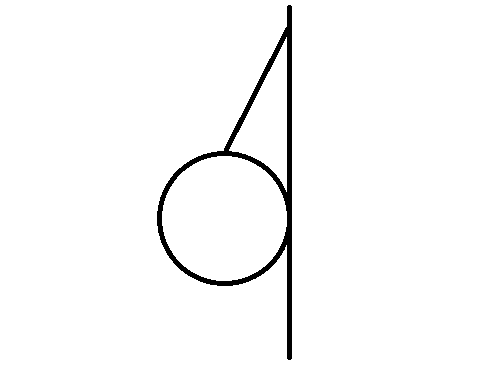# Tension of a String

• student34

## Homework Statement

A solid uniform 45.0 kg ball of radius 0.16 m is supported against a vertical, frictionless wall using a thin 0.30 m wire of negligible mass. Find the tension in the wire.## Homework Equations

Sinθ = opposite/hypotenuse

Cosθ = (m*g)/T or T/(m*g); I'm not sure

## The Attempt at a Solution

The angle is sin^(-1)(r/(r+0.30 m) = 19.471 degrees.

My textbook probably uses Cosθ = (m*g)/T to find the tension because that gives their answer OF 470.0 N. But, how can the force of the tension be greater than mg? I intuitively thought that maximum tension would be when θ = 0° and decrease to 0.00 N as θ goes to 90°.

This doesn't make any sense to me.

If you will draw a free-body diagram, you will see that the vertical component of the tension must be equal and opposite of the weight of the ball. The wire is acting at an angle to the vertical, so the tension in the wire must be greater than the vertical component.

If you were to support the ball from the ceiling such that the string was at right angles to the ceiling, then the tension would equal the weight of the ball exactly.

If you were to suspend the ball from a wire running horizontally when it is not loaded, you would see that there is a large tension in the wire required to support even a small weight. This is why overhead power lines are susceptible to breaking if they ice up.

If you will draw a free-body diagram, you will see that the vertical component of the tension must be equal and opposite of the weight of the ball. The wire is acting at an angle to the vertical, so the tension in the wire must be greater than the vertical component.

If you were to support the ball from the ceiling such that the string was at right angles to the ceiling, then the tension would equal the weight of the ball exactly.

If you were to suspend the ball from a wire running horizontally when it is not loaded, you would see that there is a large tension in the wire required to support even a small weight. This is why overhead power lines are susceptible to breaking if they ice up.

Oh yeah, thanks!